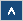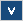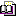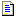Verilog Online HelpTable of ContentsBit-selectBlock StatementsBuilt-in PrimitivesCase StatementContinuous AssignmentsConversion FunctionsCommentsCompiler DirectivesConcatenationsConditional OperatorDelaysDisable StatementDisplay TasksEdge Sensitive PathExpression Bit LengthFile I/O FunctionsFunctionsIdentifiersIf StatementInteger ConstantsIntra-assignment Timing ControlsKeywordsLoop StatementsMemoriesmin:typ:max DelaysModule DeclarationModule InstantiationModule Path DeclarationModule Path PolarityNet Data TypesOperatorsParametersPart-selectPLA Modeling TasksProbabilistic Distribution FunctionsProcedural AssignmentsProcedural Continuous AssignmentsProcedural Timing ControlRange SpecificationReal ConstantsRegister Data TypesSimulation Control TasksSimulation Time FunctionsSpecify BlockState Dependent PathStochastic Analysis TasksStrengthsStringsStructured ProceduresTasksTimescale System TasksTiming Check TasksUDP DeclarationUDP InstantiationUDP State TableValue Change Dump (VCD) FileVectors

## Formal Definition

The PLA system tasks are used to model PLA devices.

## Simplified Syntax

\$array_type\$logic\$format (memory_name, input_terms, output_terms) ;

array_type ::= sync | async

logic ::= and | or | nand | nor

format ::= array | plane

memory_name ::= identifier

input_terms ::= {scalar_variable}

output_terms ::= {scalar_variable}

## Description

These system tasks are used to model content addressed memories i.e., memories that are read at locations that an input address matches a stored address. The PLA devices can be synchronous or asynchronous (type is defined by array_type: sync and async).

Both the input_terms and the output_terms should be concatenations of scalar variables, but all variables from the output_terms should be declared as a reg data type. The input_terms should have the length equal to the memory word size. The output_terms should have the length equal to the number of memory words (Example 1).

The input data is taken from the input_terms and then compared with all patterns from memory. If any pattern from the memory matches the input data then the corresponding bit of the output_terms becomes '1'.

The format specifies the logic type of an array. If an array is used, then only the bits with '1' value from the pattern are compared with the input data. If plane is used, then both '0' and '1' values are significant. The don't care values can also be specified by 'z' and '?' characters. (Example 3).

The logic identifier specifies how the input data should be compared with patterns (it also depends on the logic type of an array). If there is an and identifier then all bits with '1' value (array type) or all bits with '0' and '1' value (plane type) from the pattern should match the corresponding bits from the input data (Example 2). If a or identifier is used then at least one bit with '1' value (array type) or at least one bit with '0' or '1' value (plane type) from the pattern should match the corresponding bit from the input data.

The contents of a memory can be changed during the simulation.

## Examples

Example 1

module pla(a0, a1, a2, a3, a4, a5, a6, a7, b0, b1, b2);
input a0, a1, a2, a3, a4, a5, a6, a7;
output b0, b1, b2;
reg b0, b1, b2;
reg [7:0] mem[0:2];
initial begin
mem = 8'b11001100;
mem = 8'b00110011;
mem = 8'b00001111;
\$async\$and\$array(mem, {a0,a1,a2,a3,a4,a5,a6,a7}, {b0,b1,b2});
end
endmodule

The PLA device has an 8-bit input ([7:0]) bus and a 3-bit output ([0:2]) bus declared as reg (b0, b1, b2). This PLA device is an asynchronous array declared with logic and.

The simulation results for the PLA device from Example 1 are as follows:

A = {a0, a1, a2, a3, a4, a5, a6, a7}
B = {b0, b1, b2}
mem = 8'b11001100;
mem = 8'b00110011;
mem = 8'b00001111;
A = 11001100 -> B = 100
A = 00110011 -> B = 010
A = 00001111 -> B = 001
A = 10101010 -> B = 000
A = 01010101 -> B = 000
A = 11000000 -> B = 000
A = 00111111 -> B = 011

All '1's from the pattern should match the '1's from the input data (the input data can also have other '1's)

The simulation results for the PLA device from Example 1 declared with \$async\$and\$plane are as follows:

A = {a0, a1, a2, a3, a4, a5, a6, a7}
B = {b0, b1, b2}
mem = 8'b11001100;
mem = 8'b00110011;
mem = 8'b00001111;
A = 11001100 -> B = 100
A = 00110011 -> B = 010
A = 00001111 -> B = 001
A = 10101010 -> B = 000
A = 01010101 -> B = 000
A = 11000000 -> B = 000
A = 00111111 -> B = 000

All '0's and '1's from the pattern should match the '0's and '1's from the input data.

If a PLA is declared with \$async\$and\$plane then the pattern can be specified as 8'b0011????.

8'b0011???? -> ~a & ~a & a & a

The value of a, a, a, and a bits are not important (the don't care values specified by '?' character).

## Important Notes

• If more than one pattern matches the input data then more than one output bit will go to '1'.